### envexample1.R

maechler — Mar 3, 2014, 11:57 PM

``````##__ Original : Norm Matloff's  "Art of R ..."  Code/Ch7/envexample1.R

###--- Lexical Scoping ("Syntactic": *where* is the function defined) -----------------------
##
## h() finds 'd' inside f()  and finds 'w'   in  globalenv()
f <- function(y) {
h <- function() {
return(d*(w+y))
}
d <- 8
## h's environment is the local environment() of f(); ==> also contains 'd'

print(environment(h))
cat("environment(h):              "); print(ls(environment(h)))
cat("inside f - the same as '(h)':"); print(ls(environment()))
cat("environment(f) [=globalenv]: "); print(ls(environment(f))) # = Globalenv

return(h())
}

w <- 10
f(3)
``````
``````<environment: 0x28e6b38>
environment(h):               "d" "h" "y"
inside f - the same as '(h)': "d" "h" "y"
environment(f) [=globalenv]:  "f"        "Mlibrary" "w"
``````
`````` 104
``````
``````rm(w)
## Sometimes we think we rather "want"  **dynamic scoping**
## but R uses  "only" syntactic scoping :
g <- function(a) {
w <- 10
f(a)
}

g(3) ## --> error:
``````
``````<environment: 0x2a86de0>
environment(h):               "d" "h" "y"
inside f - the same as '(h)': "d" "h" "y"
environment(f) [=globalenv]:  "f"        "g"        "Mlibrary"
``````
``````Error: object 'w' not found
``````
``````
## w is *not* found:  it is in caller (function)
## but neither in h or f  nor in globalenv

##------------------ Functions returning Functions ----------------
## --> the result functions are **closures**  (in a stricter sense),
## namely functions with non-trivial environment, or
##
##  Closure :=   function + data  , i.e.
##           =   function + non-trivial environment

## Useful practical example:
?splinefun
?approxfun

example(splinefun) ## runs all code from the *help* page  'Examples' section
``````
``````
splnfn> require(graphics)

splnfn> op <- par(mfrow = c(2,1), mgp = c(2,.8,0), mar = 0.1+c(3,3,3,1))

splnfn> n <- 9

splnfn> x <- 1:n

splnfn> y <- rnorm(n)

splnfn> plot(x, y, main = paste("spline[fun](.) through", n, "points"))
``````
``````
splnfn> lines(spline(x, y))

splnfn> lines(spline(x, y, n = 201), col = 2)

splnfn> y <- (x-6)^2

splnfn> plot(x, y, main = "spline(.) -- 3 methods")
``````
``````
splnfn> lines(spline(x, y, n = 201), col = 2)

splnfn> lines(spline(x, y, n = 201, method = "natural"), col = 3)

splnfn> lines(spline(x, y, n = 201, method = "periodic"), col = 4)
``````
``````Warning: spline: first and last y values differ - using y for both
``````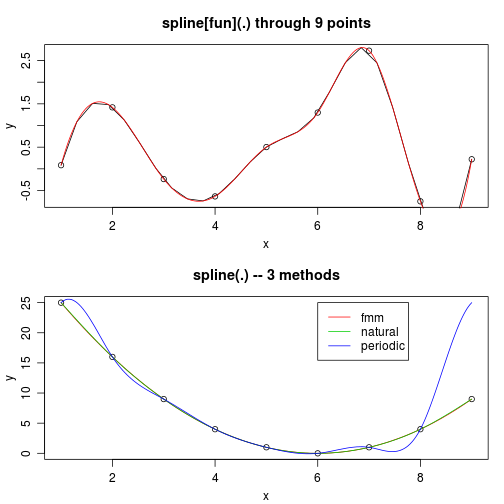``````
splnfn> legend(6, 25, c("fmm","natural","periodic"), col = 2:4, lty = 1)

splnfn> y <- sin((x-0.5)*pi)

splnfn> f <- splinefun(x, y)

splnfn> ls(envir = environment(f))
 "z"

splnfn> splinecoef <- get("z", envir = environment(f))

splnfn> curve(f(x), 1, 10, col = "green", lwd = 1.5)
``````
``````
splnfn> points(splinecoef, col = "purple", cex = 2)

splnfn> curve(f(x, deriv = 1), 1, 10, col = 2, lwd = 1.5)
``````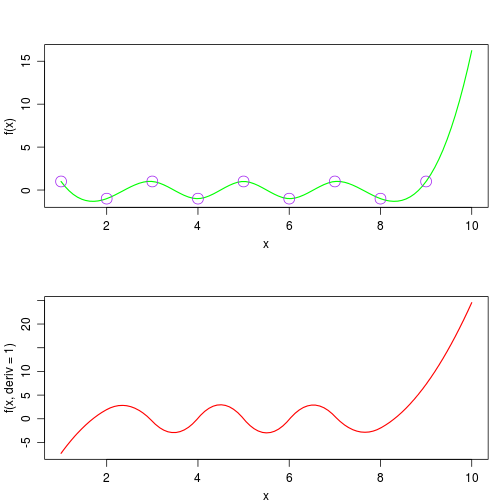``````
splnfn> curve(f(x, deriv = 2), 1, 10, col = 2, lwd = 1.5, n = 401)
``````
``````
splnfn> curve(f(x, deriv = 3), 1, 10, col = 2, lwd = 1.5, n = 401)
``````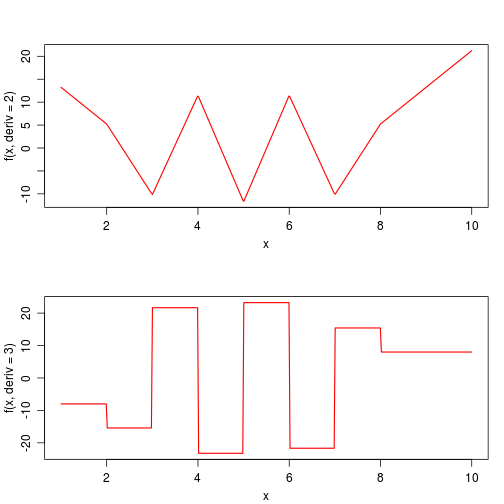``````
splnfn> par(op)

splnfn> ## Manual spline evaluation --- demo the coefficients :
splnfn> .x <- splinecoef\$x

splnfn> u <- seq(3, 6, by = 0.25)

splnfn> (ii <- findInterval(u, .x))
 3 3 3 3 4 4 4 4 5 5 5 5 6

splnfn> dx <- u - .x[ii]

splnfn> f.u <- with(splinecoef,
splnfn+             y[ii] + dx*(b[ii] + dx*(c[ii] + dx* d[ii])))

splnfn> stopifnot(all.equal(f(u), f.u))

splnfn> ## An example with ties (non-unique  x values):
splnfn> set.seed(1); x <- round(rnorm(30), 1); y <- sin(pi * x) + rnorm(30)/10

splnfn> plot(x, y, main = "spline(x,y)  when x has ties")
``````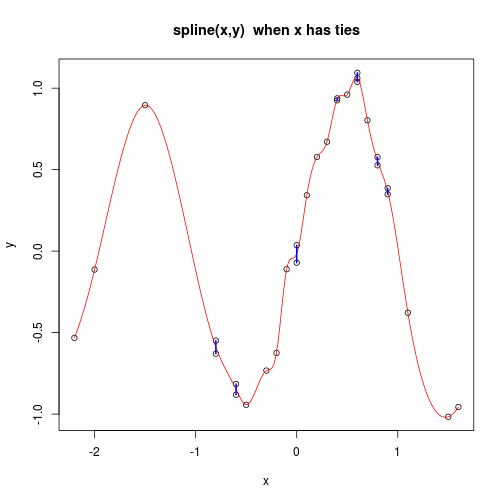``````
splnfn> lines(spline(x, y, n = 201), col = 2)

splnfn> ## visualizes the non-unique ones:
splnfn> tx <- table(x); mx <- as.numeric(names(tx[tx > 1]))

splnfn> ry <- matrix(unlist(tapply(y, match(x, mx), range, simplify = FALSE)),
splnfn+              ncol = 2, byrow = TRUE)

splnfn> segments(mx, ry[, 1], mx, ry[, 2], col = "blue", lwd = 2)

splnfn> ## An example of monotone interpolation
splnfn> n <- 20

splnfn> set.seed(11)

splnfn> x. <- sort(runif(n)) ; y. <- cumsum(abs(rnorm(n)))

splnfn> plot(x., y.)
``````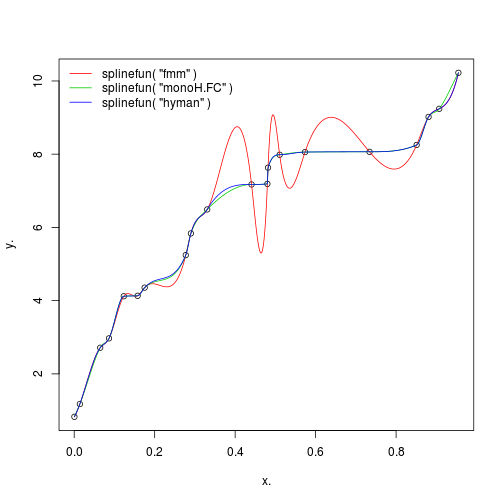``````
splnfn> curve(splinefun(x., y.)(x), add = TRUE, col = 2, n = 1001)

splnfn> curve(splinefun(x., y., method = "monoH.FC")(x), add = TRUE, col = 3, n = 1001)

splnfn> curve(splinefun(x., y., method = "hyman")   (x), add = TRUE, col = 4, n = 1001)

splnfn> legend("topleft",
splnfn+        paste0("splinefun( \"", c("fmm", "monoH.FC", "hyman"), "\" )"),
splnfn+        col = 2:4, lty = 1, bty = "n")

splnfn> ## and one from Fritsch and Carlson (1980), Dougherty et al (1989)
splnfn> x. <- c(7.09, 8.09, 8.19, 8.7, 9.2, 10, 12, 15, 20)

splnfn> f <- c(0, 2.76429e-5, 4.37498e-2, 0.169183, 0.469428, 0.943740,
splnfn+        0.998636, 0.999919, 0.999994)

splnfn> s0 <- splinefun(x., f)

splnfn> s1 <- splinefun(x., f, method = "monoH.FC")

splnfn> s2 <- splinefun(x., f, method = "hyman")

splnfn> plot(x., f, ylim = c(-0.2, 1.2))
``````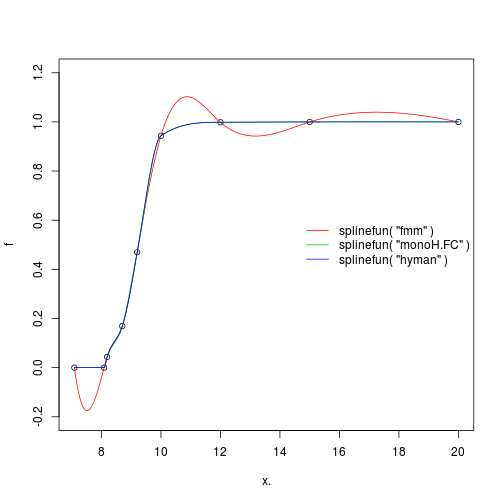``````
splnfn> curve(s0(x), add = TRUE, col = 2, n = 1001) -> m0

splnfn> curve(s1(x), add = TRUE, col = 3, n = 1001)

splnfn> curve(s2(x), add = TRUE, col = 4, n = 1001)

splnfn> legend("right",
splnfn+        paste0("splinefun( \"", c("fmm", "monoH.FC", "hyman"), "\" )"),
splnfn+        col = 2:4, lty = 1, bty = "n")

splnfn> ## they seem identical, but are not quite:
splnfn> xx <- m0\$x

splnfn> plot(xx, s1(xx) - s2(xx), type = "l",  col = 2, lwd = 2,
splnfn+      main = "Difference   monoH.FC - hyman"); abline(h = 0, lty = 3)
``````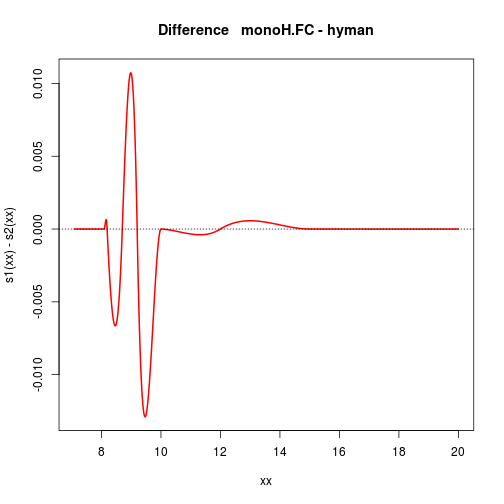``````
splnfn> x <- xx[xx < 10.2] ## full range: x <- xx .. does not show enough

splnfn> matplot(x, cbind(s0(x, deriv = 2), s1(x, deriv = 2), s2(x, deriv = 2))^2,
splnfn+         lwd = 2, col = ccol, type = "l", ylab = quote({{f*second}(x)}^2),
splnfn+         main = expression({{f*second}(x)}^2 ~" for the three 'splines'"))
``````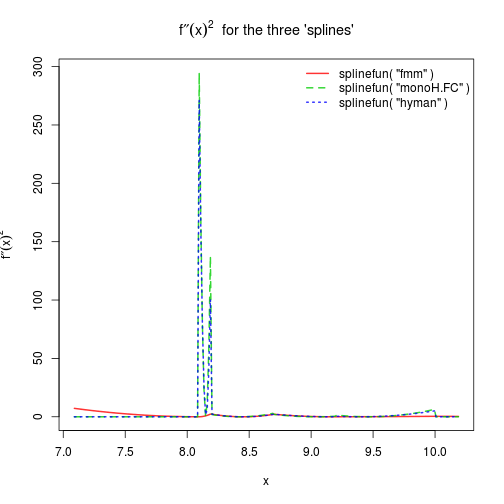``````
splnfn> legend("topright",
splnfn+        paste0("splinefun( \"", c("fmm", "monoH.FC", "hyman"), "\" )"),
splnfn+        lwd = 2, col  =  ccol, lty = 1:3, bty = "n")

splnfn> ## --> "hyman" has slightly smaller  Integral f''(x)^2 dx  than "FC",
splnfn> ## here, and both are 'much worse' than the regular fmm spline.
splnfn>
splnfn>
splnfn>
``````
``````
s0 ## prints .... last line:
``````
``````function (x, deriv = 0L)
{
deriv <- as.integer(deriv)
if (deriv < 0L || deriv > 3L)
stop("'deriv' must be between 0 and 3")
if (deriv > 0L) {
z0 <- double(z\$n)
z[c("y", "b", "c")] <- switch(deriv, list(y = z\$b, b = 2 *
z\$c, c = 3 * z\$d), list(y = 2 * z\$c, b = 6 * z\$d,
c = z0), list(y = 6 * z\$d, b = z0, c = z0))
z[["d"]] <- z0
}
res <- .splinefun(x, z)
if (deriv > 0 && z\$method == 2 && any(ind <- x <= z\$x[1L]))
res[ind] <- ifelse(deriv == 1, z\$y[1L], 0)
res
}
<bytecode: 0x2930a88>
<environment: 0x269ff48>
``````
``````## <environment: ......>   i.e., *not* the globalenv
## Looking at the function body:  where's the funny  'z'  from ??

ls( environment(s0) ) # aha, from the environment
``````
`````` "z"
``````
``````ls.str( environment(s0) )
``````
``````z : List of 7
\$ method: int 3
\$ n     : int 9
\$ x     : num [1:9] 7.09 8.09 8.19 8.7 9.2 10 12 15 20
\$ y     : num [1:9] 0.00 2.76e-05 4.37e-02 1.69e-01 4.69e-01 ...
\$ b     : num [1:9] -0.903 0.462 0.386 0.364 0.706 ...
\$ c     : num [1:9] 1.3451 0.0202 -0.7799 0.7366 -0.0532 ...
\$ d     : num [1:9] -0.442 -2.667 0.991 -0.527 -0.11 ...
``````